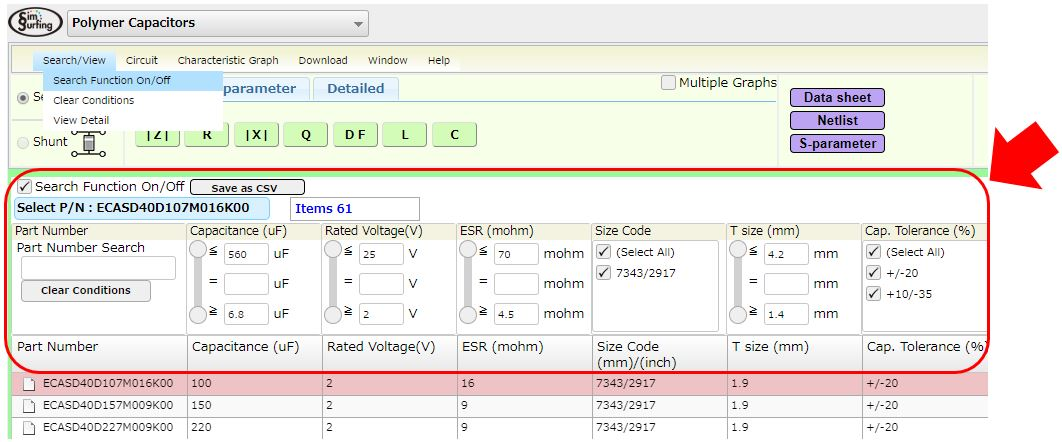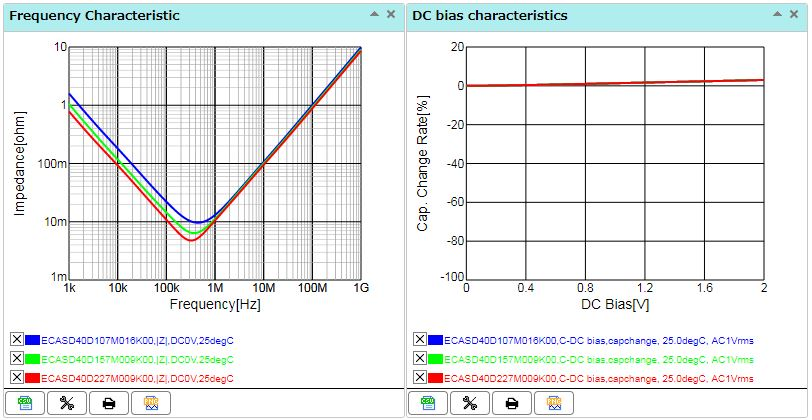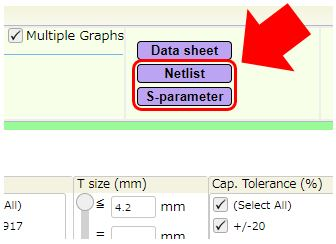# Polymer Capacitors - What can be done in SimSurfing

The following introduces what can be done in SimSurfing for polymer capacitors.

#### finding the polymer capacitors you are searching for

As shown below, multilayer ceramic capacitors can be searched from polymer capacitors.

1. Specifications: Capacitance, rated voltage, ESR, outer dimensions, etc.
2. Characteristics values: Enter to specify the impedance range / effective electrostatic capacitance, etc.
3. Murata Part Numbers#### Confirming Characteristics

The various characteristics of polymer capacitors can be displayed in graphs.

Impedance, ESR, reactance, Q, dielectric dissipation factor (tanδ), equivalent inductance, equivalent capacitance, transmission characteristics (S21), reflection characteristics (S11), DC bias characteristics, temperature characteristics, and AC voltage characteristics

In the graph, the characteristics can be compared easily, because the characteristics of multiple items can be overwritten.

* In the XY axis of the graph, the Linear/Log can be switched and the display range can be set.#### Acquiring Data used in Circuit Simulations

The S-parameters and SPICE models (Netlist) that can be used in commercial and free circuit simulations can be downloaded for the products displayed in SimSurfing.